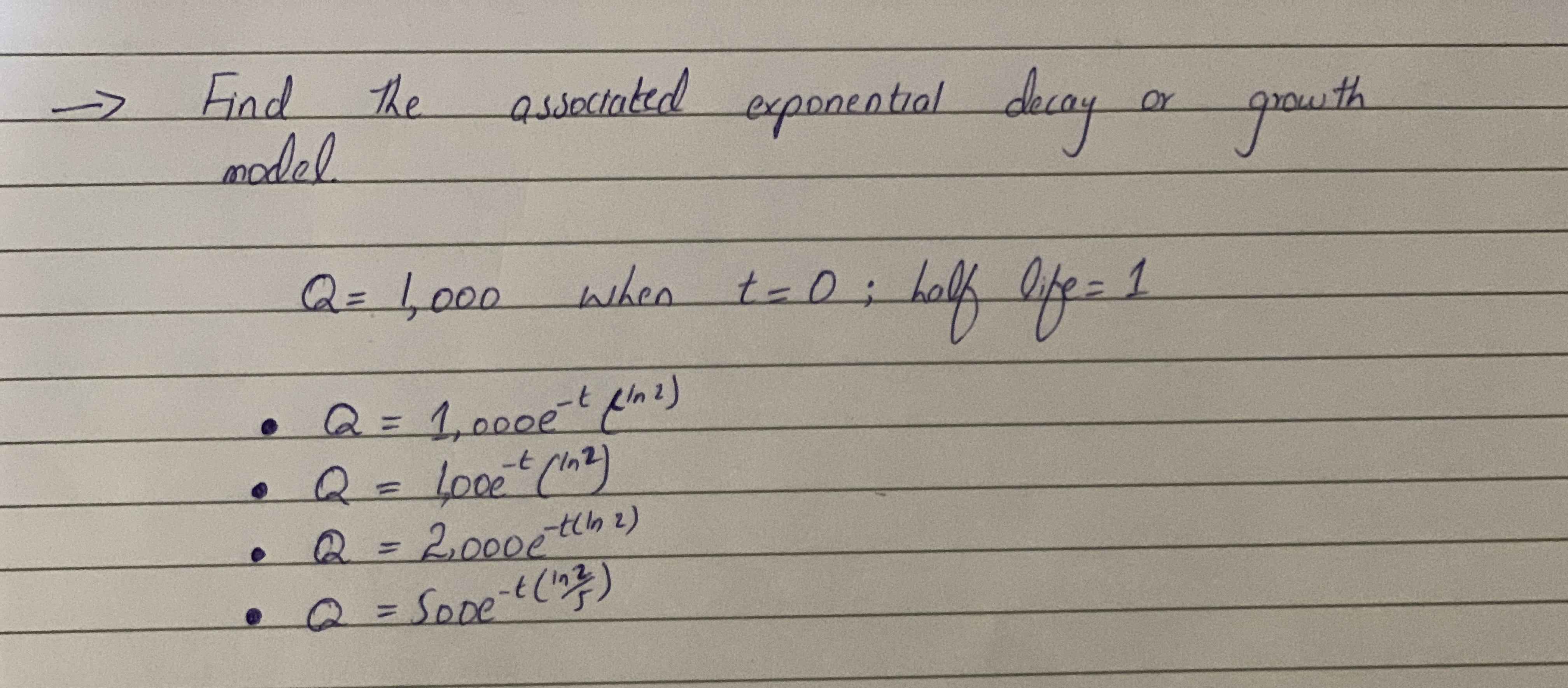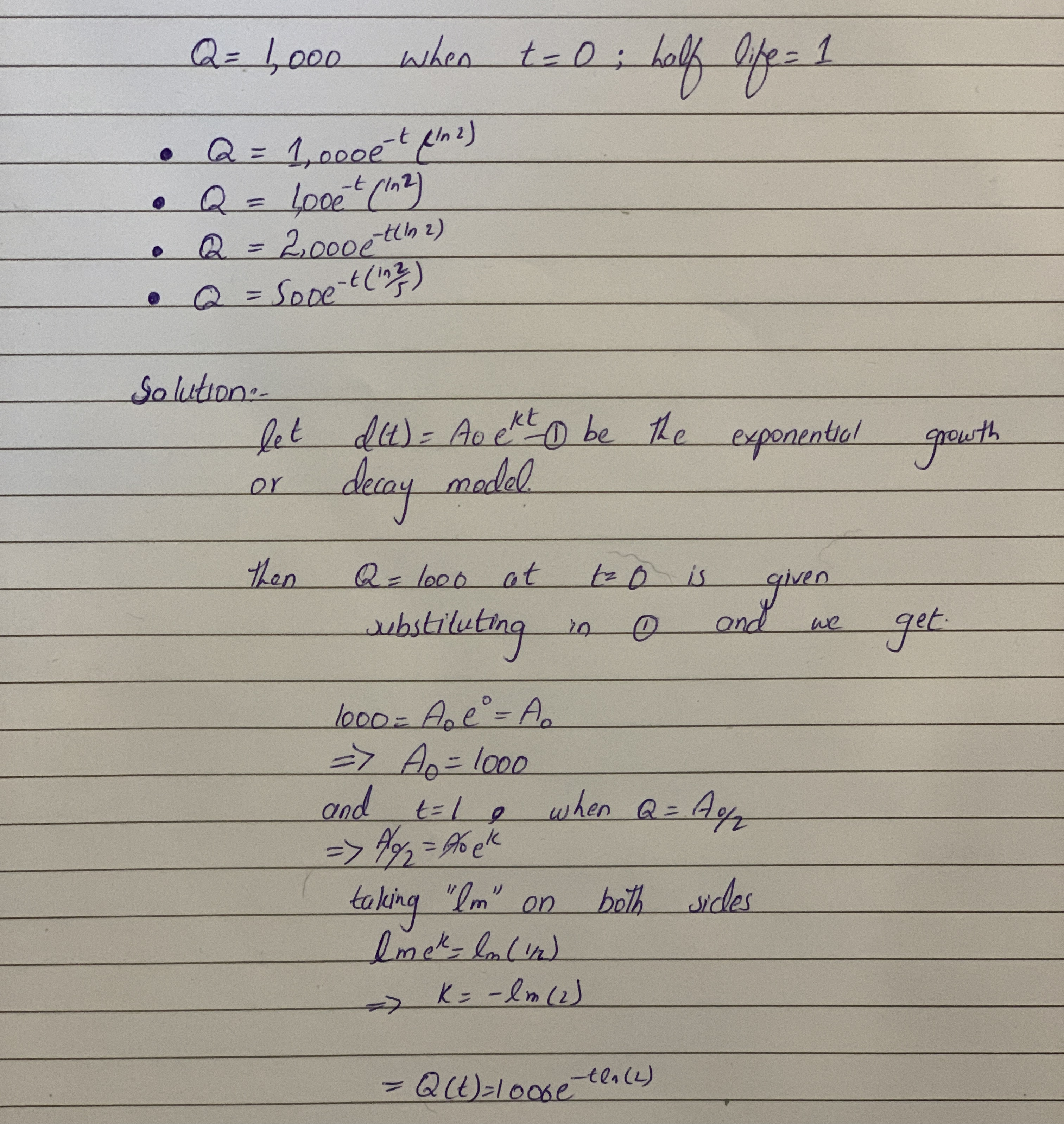# 13310200671.JPGJason Farmer 2021-02-01 Answered

Any explanation of this problem?You can still ask an expert for help

• Questions are typically answered in as fast as 30 minutes

Solve your problem for the price of one coffee

• Math expert for every subject
• Pay only if we can solve itsmallq9.

###### Not exactly what you’re looking for?user_27qwe

An exponential decay function has the form

$Q\left(t\right)={Q}_{0}{e}^{k-t}$

${Q}_{0}$ is the quantity at $t=0$.

The decay constant $k$ and half-life th for $Q$ are related by

${t}_{h}k=\mathrm{ln}2.$

Given ${t}_{h}=1$,

$1\ast k=\mathrm{ln}2$

$k=\mathrm{ln}2$

Given that ${Q}_{0}=1000$, the model is

$Q\left(t\right)=100{e}^{-t\ast \mathrm{ln}2}$